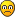## Modify only one number in array

### Modify only one number in array

How can modify a single number in array ?

Example :
I have a arraw with [3 ,1] and i want to modify only the first value to 6
Like this = [6 ,1]
Skrubs

Posts: 16
Joined: Tue Mar 06, 2018 7:36 pm

### Re: Modify only one number in array

I don't think there is an easy method for this. I just feed in the same variables from before.
Code: Select all
`someArr = [3, 1]someArr = [6, someArr(1)]`
Fisher

Posts: 2
Joined: Wed Jul 04, 2018 1:08 am

### Re: Modify only one number in array

Use the index.

Array=[3, 1]
3 : index (0)
1 : index (1)

So, use something like Array(0)=6
Now Array=[6, 1]
francky380a380

Posts: 48
Joined: Tue Dec 17, 2013 8:12 pm

### Re: Modify only one number in array

No one worked hereSkrubs

Posts: 16
Joined: Tue Mar 06, 2018 7:36 pm

### Re: Modify only one number in array

i want to make a subtraction in one array element
Skrubs

Posts: 16
Joined: Tue Mar 06, 2018 7:36 pm

### Re: Modify only one number in array

When do you want it to be modified ?
What's the action that provocs the substraction ?

Can you tell more ?
francky380a380

Posts: 48
Joined: Tue Dec 17, 2013 8:12 pm

### Re: Modify only one number in array

I want to make a subtraction in the anglevel element0 oncollide(){}
Skrubs

Posts: 16
Joined: Tue Mar 06, 2018 7:36 pm

### Re: Modify only one number in array

Write a value to a single array element.

Currently you cannot write to a single array element in the usual way that you would do it in many other programming languages. For example, you cannot do: scene.my.array(17)=2.035. So, back around 2012, one of the clever Algodoo users wrote a function that allows writing to a single array element. Here is the function code that you must first enter into the console:

//copy starts here//
scene.my.xFor := (n1, n2, code) => {
n2 > n1 ? {
m := (n1 + n2) / 2;
scene.my.xFor(n1, m, code);
scene.my.xFor(m + 1, n2, code)
} : {code(n1)}
};

scene.my.WriteArray := (array, n, new) => { result := []; scene.my.xFor(0, string.length(array)-1, (i)=>{ i == n ? { result = result ++ [new]} : {result = result ++ [array(i)]} }) }
//copy ends here//

Then in your geometry script, you call the function like this:

array=scene.my.writearray(array,n,value) where, "array" is the name of the array that you want to write to. "n" is the element number (0 is the first element). And "value" is the value that you want to write to the element. For example:

scene.my.colorarray=scene.my.writearray(scene.my.colorarray,17,3.1416)

NOTE: scene.my.xfor is a FOR loop that user Kilinich designed. The following is an example of useage:
scene.my.xFor(0,10000, (n) => {print(n)})

In order to write to an array element, you first must enter the above two functions (just copy and paste ONCE) into the Algodoo console and hit Return. Then, in a geometry, you use the scene.my.writearray function like this:

scene.my.ArrayName = scene.my.writearray(scene.my.ArrayName, array element number, new data)

Note: the "element number" can be a calculated value, or it can be a variable containing the element number.

I have been using this work-around since 2012 when I learned it. It's GREAT!Xray

Posts: 484
Joined: Sun Jun 17, 2012 6:12 am
Location: USA

### Re: Modify only one number in array

Too much ting to do one thing
Skrubs

Posts: 16
Joined: Tue Mar 06, 2018 7:36 pm

### Re: Modify only one number in array

One technique I tend to use in bigger equations is this:

Array1 = [3,1];

Array2 = Array1*[0,1]+[6,0]

Array2 is now [6,1]

However, as already mentioned, the easiest way for such small things is simply:

Array1 = [6,Array1(1)]
FRA32

Posts: 213
Joined: Wed Dec 03, 2014 9:51 pm